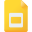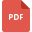# calculus tutorialsAreas Between Curves using Definite Integrals
Definite Integral, High School, United States, Calculus, Mathematics, Applications of IntegralsCurve Sketching using Calculus
Applications of Derivatives, High School, United States, Mathematics, Calculus, Curve SketchingIntegration By Parts
Integral Calculus, High School, United States, Methods of Integration, MathematicsLinear Approximations and Differentials with examples
Derivative, High School, United States, Approximations, Calculus, LinearizationVector Calculus ( 2-D and 3-D )
Vector Calculus, High SchoolHow Derivatives Affect the Shape of a Graph
Derivative, High School, United States, Differentiation, Applications of Derivatives, Calculus, MathematicsHow to Calculate Average Value of a Function using Definite Integrals
Integral Calculus, High School, United States, Applications of Integrals, MathematicsIndeterminate Forms and L’Hospital’s Rule
Applications of Derivatives, High School, United States, Differentiation, CalculusTeaching Calculus: Integration and Differentiation
Mathematics, Class 12, India, Integral CalculusGraphing with Calculus and Calculators
Calculus, High School, United States, Mathematics, GraphingThe Substitution Rule in Integration
Integral Calculus, High School, United States, Mathematics, Substitution Rule, Methods of IntegrationIntegration of Rational Functions by Partial Fractions
Methods of Integration, High School, United States, Integral Calculus, MathematicsCalculate Volume of Cylindrical Shells using Integration
Applications of Integrals, High School, United States, Integral Calculus, Definite Integral, MathematicsCalculating Work using Integration
Calculus, United States, Integral CalculusIndefinite Integrals and the Net Change Theorem
Indefinite Integral, High School, United States, Calculus, MathematicsOptimization Problems using Derivatives
Applications of Derivatives, High School, United States, Differentiation, CalculusThe Fundamental Theorem of Calculus
Calculus, High School, United States, MathematicsIntroduction to Limits
Calculus, High School, Limits and ContinuityNewton's Method to solve high degree equations
Applications of Derivatives, High School, United States, Calculus, Newton's MethodLogistic Growth Model of an Exponential Function
Logarithmic Functions, High School, United States, Integral Calculus, MathematicsStrategies for Integration
Methods of Integration, High School, United States, Integral Calculus, MathematicsSolving real life problems using differentiation
Exponential Functions, High School, United States, Derivative, Differentiation, CalculusDiffertitation Rules: Related Rates - Problems and Examples
Derivative, High School, United States, Calculus, Related RatesConcavity and the Second Derivative Test
Applications of Derivatives, High School, United States, Calculus, DifferentiationFinding Maxima and Minima using Derivatives
Differentiation, High School, United States, Calculus, DerivativeIncreasing and Decreasing Functions and the First Derivative Test
Derivative, High School, United States, Calculus, Applications of DerivativesWhat is CALCULUS? - An Introduction
Calculus, High School, United States, MathematicsHow to Calculate Exponential Growth and Decay using Calculus
Integral Calculus, High School, United States, Exponential Functions, MathematicsAntiderivatives Rules, Formulas and Examples
Antiderivative, High School, United States, Differentiation, CalculusCalculating Areas and Distances using Integrals
Integral Calculus, High School, United States, MathematicsIntegration by Substitution and Separable Differential Equations
Substitution Rule, High School, United States, Methods of Integration, Integral Calculus, Mathematics